# Cylinder (geometry)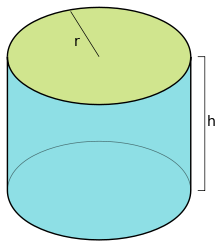A right circular cylinder with radius r and height h.

In its simplest form, a cylinder (from Greek κύλινδρος – kulindros, "roller, tumbler") is the surface formed by the points at a fixed distance from a given straight line called the axis of the cylinder. It is one of the most basic curvilinear geometric shapes.

## Common use

Commonly the word cylinder is understood to refer to a finite section of a right circular cylinder having a finite height with circular ends perpendicular to the axis as shown in the figure. If the ends are open, it is called an open cylinder. If the ends are closed by flat surfaces it is called a solid cylinder. The formulae for the surface area and the volume of such a cylinder have been known since deep antiquity.

### Volume

If the cylinder has a radius r and length (height) h, then its volume is given by

V = πr2h

Having a right circular cylinder with a height h units and a base of radius r units with the coordinate axes chosen so that the origin is at the center of one base and the height is measured along the positive x-axis. A plane section at a distance of x units from the origin has an area of A(x) square units whereorAn element of volume, is a right cylinder of base area Awi square units and a thickness of Δix units. Thus if V cubic units is the volume of the right circular cylinder, by Riemann sums,Using cylindrical coordinates, the volume can be calculated by integration over### Surface area

Still using a radius r and length (height) h, the surface area of a cylinder is made up of three parts:

• the area of the top: πr2
• the area of the bottom: πr2
• the area of the side: rh

The area of the top and bottom is always the same, and is also called the base area, B. The area of the side is also known as the lateral area, L.

An open cylinder does not include either top or bottom elements, and therefore has surface area (lateral area)

L = 2πrh.

The surface area of a closed cylinder is made up the sum of all three components: top, bottom and side. Its surface area is

A = 2πr2 + 2πrh = 2πr(r + h) = πd(r + h)=L+2B,

where d is the diameter.

For a given volume, the closed cylinder with the smallest surface area has h = 2r. Equivalently, for a given surface area, the closed cylinder with the largest volume has h = 2r, i.e. the cylinder fits snugly in a cube (height = diameter).

### Cylindric sections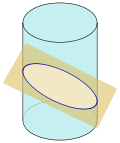Cylindric section.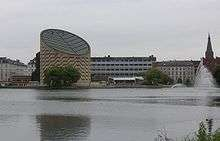Tycho Brahe Planetarium building, Copenhagen, its roof being an example of a cylindric section

Cylindric sections are the intersections of cylinders with planes. For a right circular cylinder, there are four possibilities. A plane tangent to the cylinder meets the cylinder in a single straight line segment. Moved while parallel to itself, the plane either does not intersect the cylinder or intersects it in two parallel line segments. All other planes intersect the cylinder in an ellipse or, when they are perpendicular to the axis of the cylinder, in a circle.

Eccentricity e of the cylindric section and semi-major axis a of the cylindric section depend on the radius of the cylinder r and the angle between the secant plane and cylinder axis α in the following way:## Other types of cylinders

In differential geometry, a cylinder is defined more broadly as any ruled surface spanned by a one-parameter family of parallel lines. A cylinder whose cross section is an ellipse, parabola, or hyperbola is called an elliptic cylinder, parabolic cylinder, or hyperbolic cylinder respectively.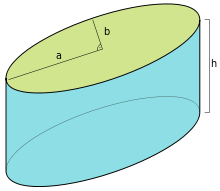An elliptic cylinder with the half-axes a and b for the surface ellipse and the height h.

An elliptic cylinder is a quadric surface, with the following equation in Cartesian coordinates:This equation is for an elliptic cylinder, a generalization of the ordinary, circular cylinder (a = b). Elliptic cylinders are also known as cylindroids, but that name is ambiguous, as it can also refer to the Plücker conoid. The volume of an elliptic cylinder with height h is. Even more general than the elliptic cylinder is the generalized cylinder: the cross-section can be any curve.

The cylinder is a degenerate quadric because at least one of the coordinates (in this case z) does not appear in the equation.

An oblique cylinder has the top and bottom surfaces displaced from one another.

There are other more unusual types of cylinders. These are the imaginary elliptic cylinders:the hyperbolic cylinder:and the parabolic cylinder:### Right circular hollow cylinder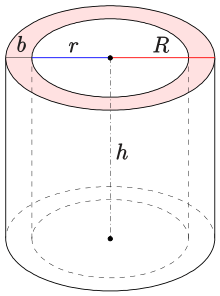Hollow cylinder(geometry)

A right circular hollow cylinder is a three-dimensional region bounded by two concentric cylindrical sections and two parallel annular bases perpendicular to the cylinder's axis.

Let the height be h, internal radius r, and external radius R. The volume is given by.

The surface area is given by.

Consider an infinite cylinder whose axis lies along the vectorWe make use of spherical coordinates:These variables can be used to define A and B, the orthogonal vectors that form the basis for the cylinder:With these defined, we may use the familiar formula for a cylinder:where R is the radius of the cylinder. These results are usually derived using rotation matrices.

## Projective geometry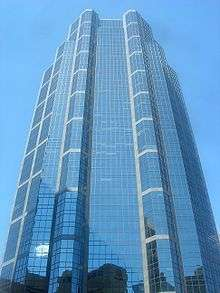In projective geometry, a cylinder is simply a cone whose apex is at infinity, which corresponds visually to a cylinder in perspective appearing to be a cone towards the sky.

In projective geometry, a cylinder is simply a cone whose apex is at infinity.

This is useful in the definition of degenerate conics, which require considering the cylindrical conics.

A cylinder can be seen as a polyhedral limiting case of an n-gonal prism where n approaches infinity. It can also be seen as a dual of a bicone as an infinite-sided bipyramid.

Polyhedron Coxeter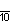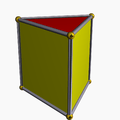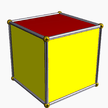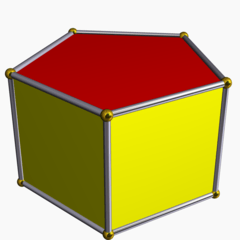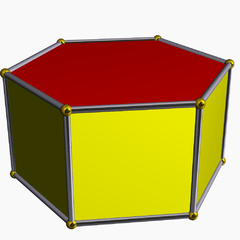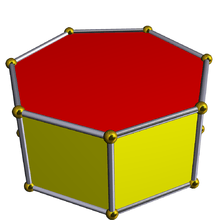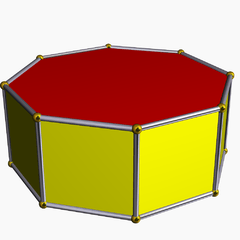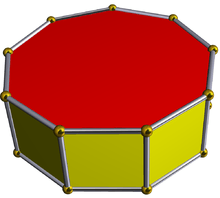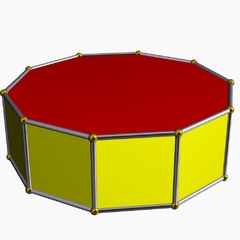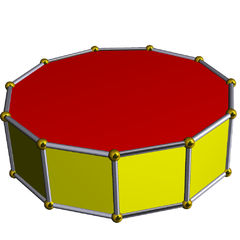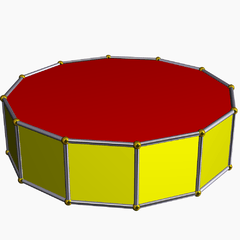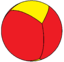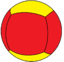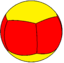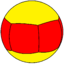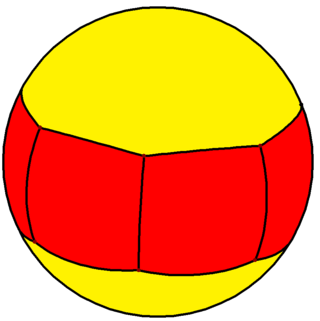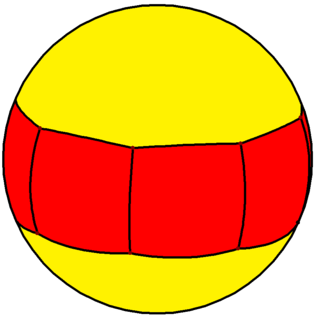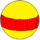Wikimedia Commons has media related to Cylinder (geometry).Wikisource has the text of the 1911 Encyclopædia Britannica article Cylinder.Look up cylinder in Wiktionary, the free dictionary.